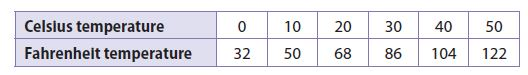Homework Explained - Math Practice 101Dear guest, you are not a registered member. As a guest, you only have read-only access to our books, tests and other practice materials.

As a registered member you can:

Registration is free and doesn't require any type of payment information. Click here to Register.
Go to page:
Chapter 3: Proportional Relationships; Lesson 1: Representing Proportional Relationships

Independent Practice

The table shows the relationship between temperatures measured on the Celsius and Fahrenheit scales.• Question 7

Is the relationship between the temperature scales proportional? Why or why not?

• Question 8

Describe the graph of the Celsius-Fahrenheit relationship.

• Type below:
• Question 9

Analyze Relationships

Ralph opened a savings account with a deposit of $100. Every month after that, he deposited$20 more.

a. Why is the relationship described not proportional?

• Type below:
• Question 9

b. How could the situation be changed to make the situation proportional?

• Type below:
• Question 10

Represent Real-World Problems

Describe a real-world situation that can be modeled by the equation $$y=\large\frac{1}{20}\small\text{x}$$. Be sure to describe what each variable represents.

• Type below:

Look for a Pattern

The variables x and y are related proportionally.

• Question 11

When x = 8, y = 20. Find y when x = 42.

• Question 12

When x = 12, y = 8. Find x when y = 12.

Yes, email page to my online tutor. (if you didn't add a tutor yet, you can add one here)